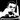# Cleaner Clojure Functions with the :pre Special Form

January 03, 2019

In the course of programming, it’s fairly common to face a scenario where you want to ensure that a function’s arguments fulfil some condition(s), and if not, to throw an error.

For example, if our function should only accept positive numbers.

In this post, we’re going to see how Clojure provides a handy special form that greatly simplifies this task.

One such scenario that I’ve come across recently is trying to come up with a function that would compute the 3x + 1 problem (Collatz conjecture). The problem is as follows:

Take any positive integer n.

If n is even, divide n by 2 to get n / 2.

If n is odd, multiply n by 3 and add 1 to get 3n + 1.

Repeat the process indefinitely.

The conjecture states that no matter which number you start with, you will always reach 1 eventually.

The task at hand was to write a function that takes a number as an argument and returns the number of steps it takes for the number to eventually be 1.

You’ll also notice that the first condition for the number to be passed in is that it has to be a positive integer. Therefore, given any value less than 1, the function should throw an error.

A typical solution in Clojure would look as follows:

``````(defn compute-collatz [num steps]
(cond
(= num 1) steps
(even? num) (compute-collatz (/ num 2) (inc steps))
(odd? num)  (compute-collatz (+ 1 (* num 3)) (inc steps))))

(defn collatz [num]
; Check if the provided number is positive. If not throw an error
(if
(pos? num) (compute-collatz num 0)
(throw (AssertionError. "Positive integer required."))))``````

While this definitely works, I found out that Clojure provides a much more elegant way to accomplish this, with the help of the `:pre` special form.

This form enables specifying conditions about the arguments that should be met before the rest of the function body is evaluated. If any of the conditions is not met, an assertion error is thrown.

This is how we could rewrite the function making use of the `:pre` condition:

``````(defn compute-collatz [num steps]
(cond
(= num 1) steps
(even? num) (compute-collatz (/ num 2) (inc steps))
(odd? num)  (compute-collatz (+ 1 (* num 3)) (inc steps))))

(defn collatz [num]
{:pre [(pos? num)]}
(compute-collatz num 0))``````

In this case, if the function is called with `0` or a negative number, an assertion error is automatically thrown.

I think you would agree that the second version looks much nicer 🙂Written by Kevin Ndung'u